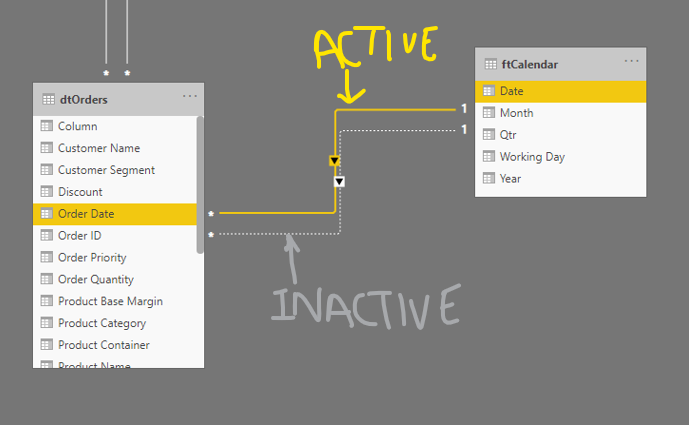top of page
Search

# Multiple Dates on the same axis: DAX USERELATIONSHIPOne of the key benefits of creating a date or calendar table in Power BI is that we can blend multiple dates on a single axis.

Let us consider the following example:We need to get the total Order Quantity by Order Date & Ship Date.

It is a three-step process:

• adding a calendar table in the data model

• establish relationships between the calendar table and fact table

• and use the DAX USERELATIONSHIP

When dealing with dates, it is best to add a calendar table in the data model. The simplest way to do it is to add a table by using the following DAX:

``` ftCalendar =
CALENDARAUTO(),
"Year", YEAR([Date]),
"Month", EOMONTH([Date],-1)+1,
"Qtr", "Q" & FORMAT([Date],"q")
)
```

You may follow the article for details on adding a date/calendar table

## Establishing Relationship

Since we are dealing with multiple dates, it requires creating multiple relationships between the fact table (in this case, the Order table) and the calendar table:In the case of multiple relationships between two tables, there can be only one active relationship (Denoted by a solid line). The rest will all be inactive. A dotted line denotes it in the Model ViewDiagram View in Power Pivot (Like Model View in Power BI Desktop)## USERELATIONSHIP

Since there is an existing relationship between Order Date and the Calendar table date, the following measure calculates the total orders by Order Date:

``` Total Orders = SUM(dtOrders[Order Quantity])
```

To calculate the sum of orders by Ship Date, we use the DAX USERELATIONSHIP

``` Orders by Ship Date =
CALCULATE(
[Total Orders],
//temporarily activating the inactive relationship
USERELATIONSHIP(ftCalendar[Date],dtOrders[Ship Date])
)
```

Essentially, we are telling DAX to calculate the sum of orders, but this time use the relationship between the Ship Date and Calendar Table date field to show the output. It temporarily activates the relationship between the two fields.

Following is the output:## Points to consider:

• We can use the DAX USERELATIONSHIP in functions that take filter as an argument, such as CALCULATE, CALCULATETABLE, TOTALMTD, TOTALQTD, TOTALYTD.

• It uses the existing relationship between the two tables. If there is no relationship, then we cannot use USERELATIONSHIP. In such cases, we can use TREATAS

• For USERELATIONSHIP, the status of the relationship is not vital, i.e., whether the relationship is active or inactive. Consider the following data model:

None of the relationships are active in the following data model:Since all the relationships are inactive, we have used the following DAX measures to get the count of employees by Joining Date (DOJ) and Last Working Date (LWD) by month:

``` Joining by Month:=
CALCULATE(
COUNTROWS(EmpMaster),
USERELATIONSHIP('Calendar'[Date],EmpMaster[DOJ])
)

Inactive by Month:=
CALCULATE(
[Inactive Employees],
USERELATIONSHIP('Calendar'[Date],EmpMaster[LWD])
)
```

Following is the output:So, in the year 2003, 10 employees joined, and 3 employees left.